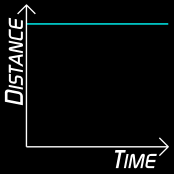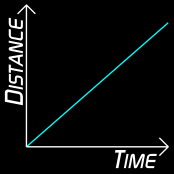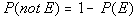Wednesday, June 24, 2009

Speed-Time Graph

Continued with the topic of distance-time graph with my 5KA girls today. We discussed a couple of questions on the topic. Most of the students were able to solve the problems given (I wish they can do the same in the next test). Then I managed to introduce the speed-time graph.

Speed-Time Graph

We can represent the movement of objects using a graph, usually plotting time on the x-axis (horizontal) and the speed on the y-axis (vertical).

Consider a train accelerating from a station along a straight and level track to a maximum speed and slowing down to a stop at the next station. The easiest way to show this is with a speed time graph.Acceleration is the gradient of the speed-time graph.

From the graph,

* between O and A, the train is accelerating;
* between A and B, the train travels at a constant speed;
* between B and C, the train slows down. Slowing down can also be called
negative acceleration, or deceleration. It is given a minus sign.

Distance is the area under the speed-time graph. To work out the total distance, we would add the areas of:

* triangle OAX;
* rectangle ABXY;
* triangle BCY.

Mathematical Reasoning

Started Chapter 4 - Mathematical Reasoning for my 4SA today. In the first part, students were introduced with the concepts of statement, quantifiers, negation and compound statements. The powerpoint presentation below was used in today's class.

Saturday, June 20, 2009

Distance-Time Graph

My course on the school management system in Ipoh was cut short by one day coz some of the participants were called for a meeting on Friday in Kuala Lumpur. It has been a fruitful 3 days here in Ipoh learning about the new system which will be installed in the school server next week. Anyway, I'm glad to be able to continue my lesson with 5 KA on Friday coz i really need to cover a lot more chapters before the trial exam. Managed to start the topic on distance-time graph.

Introduction

Information about movement can be presented in a number of ways. Data for the total distance moved at different times during movement can be recorded in a table. Alternatively, the same information can be presented in a graph. Plotting distance against time can tell you a lot about a journey.

If something is not moving, a horizontal line is drawn on a distance-time graph (dt-graph).Time is increasing to the right, but its distance does not change. It is stationary

If something is moving at a steady speed, it means we expect the same increase in distance in a given time:Time is increasing to the right, and distance is increasing steadily with time. It moves at a steady speed.

Slope and the Speed

When you look at a slope of a line on a distance-time graph you may notice how slopes can be different. The slope of the line determines the speed; the higher the slope the greater the speed, but if the slope is low then the speed is low.As you can see in the first chart, the slope is very high, this means that the car must be traveling at a great speed. In the second graph, the slope is relatively low, which means that the car is driving at a very low speed

You can learn more on distance-time graph here

More on Probability

The Form Four syllabus on probability require students to calculate the probability of a specific or single event occurring. The equations below provide the formula for calculating simple probability. The first one is written out, while the second is in symbol formProperties of Probability

Property 1Property 2Property 3Click here to do challenge exercises on probability.

Wednesday, June 17, 2009

Probability

Hi, I'm back after a two week school break. Only managed to teach probability for my 4 SA class on Monday coz I've to be in Ipoh for the rest of the week for a course on the new Smart School Management System. The Probability topic for Form 4 is a very short and simple topic

The study of probability helps us figure out the likelihood of something happening. For instance, when you roll a pair of dice, you might ask how likely you are to roll a seven. In math, we call the "something happening" an "event.

Definitions :

The sample space is a set consisting of all the possible outcomes of an event (like drawing a marble from a jar, or picking a card from a deck). The number of different ways you can choose something from the sample space is the total number of possible outcomes.

Because each probability is a fraction of the sample space, the sum of the probabilities of all the possible outcomes equals one.

An experiment is a situation involving chance or probability that leads to results called outcomes.

An outcome is the result of a single trial of an experiment.

An event is one or more outcomes of an experiment.

Probability is the measure of how likely an event is.